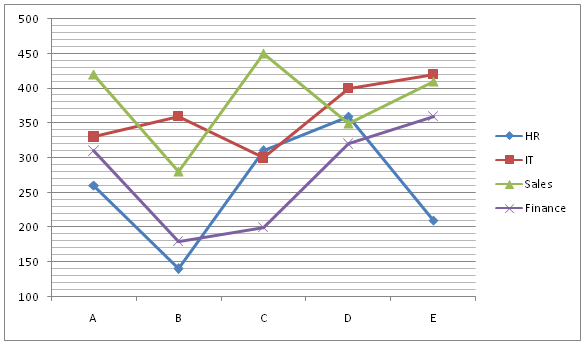# SBI Clerk Prelims 2021 Quantitative Aptitude Questions (Day-39)

Dear Aspirants, Our IBPS Guide team is providing new series of Quantitative Aptitude Questions for SBI Clerk Prelims 2020 so the aspirants can practice it on a daily basis. These questions are framed by our skilled experts after understanding your needs thoroughly. Aspirants can practice these new series questions daily to familiarize with the exact exam pattern and make your preparation effective.

Start Quiz

Missing number series

Directions (01-05): What value should come in the place of (?) in the following number series?

1) 18, 19, 39, 118, 473, ?

A.946

B.1319

C.2366

D.1893

E.2839

2) 3, 122, 224, 309, ?, 428

A.377

B.386

C.383

D.372

E.389

3) 13, 36, 65, 96, 133, ?

A.156

B.168

C.148

D.174

E.181

4) 11, 37, 76, 128, 193, ?

A.268

B.271

C.276

D.282

E.289

5) 15, 33, 54, 78, 105, ?

A.128

B.130

C.132

D.135

E.124

Line graph

Directions (06-10): Study the following information carefully and answer the questions given below.

The given line graph shows the number of women employees in four different departments in five different companies.6) What is the difference between the women employees from HR and IT department together in A and C?

A.10

B.20

C.30

D.40

E.35

7) What is the approximate average number of women employees in D from all the departments together?

A.358

B.362

C.366

D.370

E.345

8) What is the ratio of the average number of women employees from Finance to Sales department in all the companies together?

A.137:189

B.133:190

C.135:191

D.137:191

E.None of these

9) If the ratio of the number of women to men employees in B from HR and IT department is 7:5 and 3:2 respectively, then what is the difference between the number of men employees from IT and HR departments in B?

A.120

B.150

C.110

D.130

E.140

10) The number of HR women employees from all the companies together is approximately what percent of the number of IT women employees from all the companies together?

A.71%

B.73%

C.75%

D.77%

E.79%

18 * 1 + 1 = 19

19 * 2 + 1 = 39

39 * 3 + 1 = 118

118 * 4 + 1 = 473

473 * 5 + 1 = 2366

3 + (7 * 17) = 122

122 + (6 * 17) = 224

224 + (5 * 17) = 309

309 + (4 * 17) = 377

377 + (3 * 17) = 428

13 + 23 = 36

36 + 29 = 65

65 + 31 = 96

96 + 37 = 133

133 + 41 = 174

11 + (13 * 2) = 37

37 + (13 * 3) = 76

76 + (13 * 4) = 128

128 + (13 * 5) = 193

193 + (13 * 6) = 271

15 + 18 = 33

33 + 21 = 54

54 + 24 = 78

78 + 27 = 105

105 + 30 = 135

Number of women employees from IT and HR in A = 260 + 330 = 590

Number of women employees from IT and HR in C = 310 + 300 = 610

Required Difference = 610 – 590 = 20

Required average = (360 + 400 + 350 + 320)/4

= 357.5

Required ratio = (310 + 180 + 200 + 320 + 360)/5 : (420 + 280 + 450 + 350 + 410)/5

= 274:382

= 137:191

Number of men employees from HR = 5/7 * 140 = 100

Number of men employees from IT = 2/3 * 360 = 240

Difference = 240 – 100 = 140